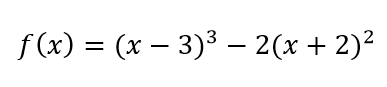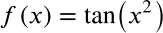Exit Ticket for Day 29 of Calculus
Please select your name from the following list: *
For the following problems, work this equation:1. What is the derivative of the expression shown above?
2. If I told you to find the "critical points" of that function, how would you do it?
3. Find the 2 critical points of the shown shown above.
4. If you knew that some value of x was a "critical point" of the function f(x), how could you determine whether that x was a RELATIVE MAXIMUM or a RELATIVE MINIMUM of the function f(x)?
5. If you look back at question 3, you'll notice that all of the options that I gave you have one of the two "critical points" of the function occurring at x=1. Therefore, you KNOW there is a critical point at that location. Is x=1 a RELATIVE MAXIMUM or a RELATIVE MINIMUM of this function?
These are review questions!
6. Find the derivative of the following function:7. Suppose we know that f(1)=17 and f(10)=17. According to Rolle's theorem, we now also know _______.
This form was created inside of Evansville Vanderburgh School Corporation. Report Abuse - Terms of Service